# Equations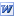Rearranging Equations Rearrange these equations to make the given terms the subject. All formulae with real applications. Harder equations where the subject appears twice are on the second page, these type of questions usually occur towards the higher end of a GCSE paper. PDF here.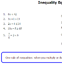Inequality Equations Some straightforward equations, some involving multiplying & dividing by -ves and some quadratics.Further Factorising Expressions of the format quadratic numerator, quadratic denominator to factorise and cancel down. PDF here.Algebra Rectangles Use the parallel sides property of rectangles to work out the missing expressions. (Thanks Hunneyball!)Equations & More A selection of linear equations to solve plus a few other questions too.Solving Equations 1 Intro worksheet to solving equations. Good for younger pupils to gain confidence in algebra. Includes Answers. PDF here.Solving Equations 2 (Unknowns Both Sides) 28 equations of progressive difficulty PDF here.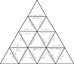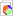Solving Equations Tarsia 15 questions to practise solving equations; all linear, some one and two step, others with unknowns on both sides. The Tarsia program for Windows is license free. Template for students to create their own.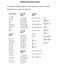Equations Game Pupils earn points by solving the equations. Easier questions earn less points so pupils decide the correct level of difficulty for themselves. Pupils with most points after a given time period win a prize? Answers to the equations are included. PDF here. (An easier version with no negative answers. PDF here.)Equations (Unknowns Both Sides) Some equations with the unknown on both sides for you to solve. No answers, sorry! PDF here.Graphs, Algebra, Number Machines (and input/output tables & words) Pupils consolidate understanding of straight line graphs by cutting up and then matching each line graph to its equation, sentence, input/output table and number machine. PDF here.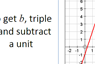Relationships A number machine, algebra, graph, sentence, sequence and input/output table for students to investigate... and hopefully come to the conclusion that all represent the same relationship in different ways. Suggestions for ways to investigate are on slide 4. PDF here. To extend this rich open task students could create their own set of cards. Lesson plan. PDF here. A subsequent activity is for students to complete the number machine, table, sentence and graph from the algebra. Prompt cards for this activity (PDF here) with lesson plan. PDF here.Circles Equations Worksheet to check understanding of association of graph of circle to its equation.